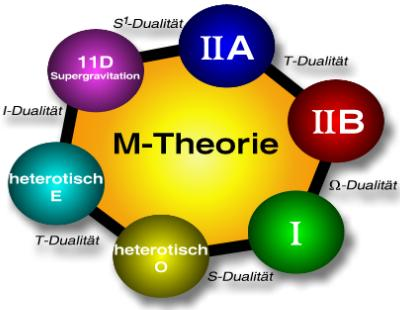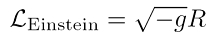# String theory predicts supersymmetry

## String theories

### Superstring Theories: supersymmetric string theories

All five string theories are super symmetric. For this reason they are also called Superstring theories. Supersymmetry (SUSY) avoids the above-mentioned tachyonic excitation states that lead to acausalities.
SUSY rules between two groups of particles: the bosons and the fermions. Every boson has a fermionic super partner, and every fermion has a boson super partner. The consequence of this is that far more particles must exist than are known in the Standard Model. So the bosonic graviton would have the fermionic super partner Gravitino or the gauge bosons as fermionic super partners Gauginos (Description of the suffixes under SUSY). All of these supersymmetric partners have relatively large masses. That is why they have not yet been discovered in particle accelerators or other experiments. Indeed, that is troubling so far not a single SUSY particle detected experimentally became - neither directly nor indirectly! Only the observation that the coupling constants of the four interactions, which is a measure of the strength of the respective force, energy dependent and approach each other at high energies, indicates the existence of SUSY.

### What do the string theories do?

• Each of the five string theories contains the Gravity, i.e. a description of gravity on the quantum level (quantum gravity) is possible.
• The string theories say one super symmetrical nature ahead. So far this property has not been confirmed experimentally, but if that is the case (possibly soon in modern particle accelerators), that would speak strongly in favor of the superstring theories. SUSY is viewed by most particle physicists as a reasonable extension of the Standard Model of particle physics.
• With the string theories, calculations can be carried out in the regime of strong coupling of quantum chromodynamics, where the perturbation theory of conventional field theories fails. With these QCD strings For example, the experimental data of gold-gold collisions measured at the RHIC particle accelerator can be explained very well.
• At the end of the 1990s, one of the most important recent findings in theoretical physics was discovered within the framework of string theories: the so-called AdS / CFT correspondence. This correspondence exists between a string theory on a 5D anti-de-sitter spacetime (abbreviated AdS), which takes gravity into account, and a conformal field theory (engl. conformal field theory, abbreviated CFT) without gravity, which only operates on the 4D edge. The 5D strings now have a number of the properties of the 4D QCD (Yang Mills Theory) to describe. It has even been possible to generalize this duality for other spacetime: it then turns out to be a fundamental one Duality between calibration and gravity (gauge / gravity dual).
• With the methods of string theories, the Bekenstein-Hawking entropy can be calculated. The result agrees with that of the classical theory of black holes. This parallel alone does not mean much; but it can be extremely interesting Analogies between quantum chromodynamics and black holes draw: A transition into a quark-gluon plasma, as described in the 4D-QCD, corresponds phenomenologically to the creation of a black hole in the 5D string theory (Maldacena, Nature 423, 695, 2003). The Bekenstein-Hawking entropy of classic black holes is then related to the entropy of a plasma made up of quarks and gluons! These are completely new insights into nature.### M-Theory - mysterious superstructure of six theories

In addition to the five string theories, physicists are familiar with the (apparently) independent supergravity, which links supersymmetry and general relativity (GTR). After 1995, the string theorists discovered that the five string theories related to each other via certain mathematical relations, the Dualities, being related (Horava & Witten 1996). The researchers suspected that there was an overarching theory of higher dimensions that contained all string theories: the eleven-dimensional M theory. M. stands for optionally for magic, mystery or matrix - all properties that are ascribed to the M-theory. Amazingly, it turned out that the M-theory contains another theory: the also eleven-dimensional supergravity (SUGRA). SUGRA and M-theory agree for small energies, i.e. supergravity is the low-energy limit of M-theory (Horava & Witten 1996). The figure above summarizes the five string theories and SUGRA to form the M-theory. To date, only a few properties of the M-theory are known. The string theorists only know that they do

• be eleven dimensional,
• correspond to supergravity in the Limes of small energies,
• their objects that M-branes, Be strings and p-branes,
• it is not perturbative, i.e. not accessible from a theoretical point of view.

If at least two strings interact with one another, a physical quantity comes into play, theCoupling constant called. The numerical accessibility of string theories depends sensitively on this quantity, because one uses the apparatus of perturbation calculus. If the coupling constant is less than one, a perturbative theory (perturbative) Treatment possible. If it is greater than one, there are, in rare exceptional cases, alternative methods. This makes the formulation and experimental verification of the M-theory so extremely difficult.

### Where are the other dimensions of space?

An interesting question is why the six additional spatial dimensions that each of the five ten-dimensional string theories has more than the four-dimensional space-time of GTR do not appear in everyday life or in physical measurements. Answer: They are 'very small', or as physicists say they are compacted. Compactification means that spatial dimensions are 'rolled up' on small spatial scales. Therefore, they usually remain hidden. You can imagine that, for example, a drawn line that appears one-dimensional to us as an observer reveals additional dimensions when magnified with a microscope: the line appears thicker, extended and becomes a two-dimensional strip.
In the ten-dimensional string theories, one examines certain subspaces with less than ten dimensions that are flat. flat) or curved curved or warped) could be. This includes the Orbifolds, Manifolds that have singular points and Calabi-Yau manifolds, the Ricci flat (the Ricci tensor vanishes) and the type Kähler metrics are. These subspaces are spanned by compacted spatial dimensions. In general, all string theories and eleven-dimensional supergravity can be broken down into submanifolds: Type II string theories correspond to a decomposition into the previously known four-dimensional space-time and three-dimensional Calabi-Yau manifold. The heterotic string theories can be broken down into four-dimensional space-time as well as a K3 surface and a two-dimensional torus. Finally, the eleven-dimensional supergravity is 'synthesized' from four-dimensional space-time, three-dimensional Calabi-Yau manifold and a one-dimensional circle.
A Soliton is a certain type of wave, a Wave packetthat spreads particularly stably. These waves, which are very resistant to interference, exist in many areas of physics, e.g. in optics (optical soliton), as well as in acoustics and hydrodynamics (Soliton water wave). All p-branes of string theories that are solitons can be found as solutions of an eleven-dimensional supergravity.
The world of ART is four-dimensional. The other seven dimensions of supergravity or M-theory are compacted. The physicists also refer to the new dimensions as Extra dimensionsthat could not be proven experimentally so far. At short distances one expects that Newton's law of gravity with its typical r2-Drop for the gravitational force Not is more valid. The physicists check this in Cavendish experiments and other tests. So far they found it no signs of further room dimensions except for the classic three. The string theorists explain this in such a way that the extra dimensions on even smaller distances be compacted than can be tested in experiments. The current limit is in the micrometer range, i.e. the so-called Compactification radius must be smaller than a millionth of a meter.
Even in modern particle accelerators, it might be possible to count the extra dimensions, namely if the experimenters there artificially create a black hole in miniature and observe its decay using Hawking radiation. The string theories could explain (but they do not necessarily require it!) If the fundamental Planck scale is reduced and therefore effects of quantum gravity could already occur on the TeV scale (TeV quantum gravity). Then particle accelerators like the LHC would be able to make these effects visible.

### String landscape: blooming landscapes of the string world

The formulation of string theories on different Calabi-Yau spaces can Mirror symmetries so that the study of one string theory reveals something about the corresponding dual mirrored string theory.
A general problem is that it is possible to choose from a large number of Calabi-Yau rooms. However, any number of ground states follow from this (metastable vacuums) in string theories. This enormous number of false vacuums is covered by the term String theory landscape (engl. string theory landscape) summarized.

### new black holes in string theories

It is even possible to generalize the classical black holes of GTR in the Branen formalism. The point-like black holes would accordingly be 0-branes, their generalizations in the form of a 1-brane, the Black string (engl. black string) and the 2-brane, the Black bran (engl. black brane) existed. As in quantum field theory, the equations of motion or field equations or Euler-Lagrange equations follow from the applied Lagrangian (Lagrangian). The classic Hilbert-Einstein long-range density (noted in the equation on the right)leads to an equation of motion (eom, engl. equation of motion), which dictated the disappearance of the Ricci tensor. So this equation has Ricci flat solutions, e.g. uncharged black holes (Schwarzschild solution). If, on the other hand, the Einstein-Maxwell Lagrangian, which contains the Maxwell tensor, is used, one finds brane generalizations of the classic Reissner-Nordstrøm solution.
A corresponding generalization of the approach for the Einstein-Maxwell Lagrangian in eleven dimensions lead to solutions that one pp wave (a certain type of gravitational wave) andDeaf NUT solution is called. The latter is a non-diagonal metric that is not asymptotically flat. The strange name of this vacuum solution of Einstein's field equations goes back to the relativists who first found them: AH. Deaf 1951 and in an expanded form E.T. Newman, Unti asTamborino 1963. This solution has nothing to do with a 'deaf nut'.
Black holes can get through too Vibrational modes of 3-branes be represented. They then carry mass, electrical charge and angular momentum. This corresponds to the Kerr-Newman solution of black holes. The amazing result is that string theory predicts exactly the same entropy as found as a thermodynamic analogue in black hole theory, namely Bekenstein-Hawking entropy.
Within the framework of the D-brane formalism, the Hawking radiation can be described: Interacting open strings on a D-brane lead to the emission of closed strings. This treatment provides what is called a Gray body spectrum (engl. gray-body spectrum), an extension of the classic black body or Planck spectrum, which is extended by an energy-dependent absorption cross-section.